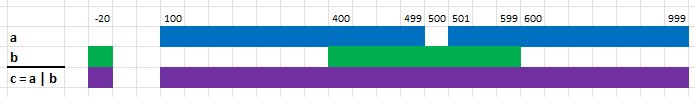## no-std range-set-blaze

Integer sets as fast, sorted, integer ranges with full set operations

### 10 releases

 0.1.9 Jun 29, 2023 Jun 29, 2023 May 23, 2023 Apr 12, 2023

#79 in WebAssembly

Used in 3 crates (2 directly)

MIT/Apache

10MB
3K SLoC

Contains (Zip file, 13KB) docs/Examples.xlsx

# range-set-blaze

Integer sets as fast, sorted, integer ranges with full set operations

The integers can be any size (`u8` to `u128`) and may be signed (`i8` to `i128`). The set operations include `union`, `intersection`, `difference`, `symmetric difference`, and `complement`.

The crate's main struct is `RangeSetBlaze`, a set of integers. See the documentation for details.

Unlike the standard `BTreeSet` and `HashSet`, `RangeSetBlaze` does not store every integer in the set. Rather, it stores sorted & disjoint ranges of integers in a cache-efficient `BTreeMap`. It differs from other interval libraries -- that we know of -- by offering full set operations and by being optimized for sets of clumpy integers.

We can construct a `RangeSetBlaze` from unsorted & redundant integers (or ranges). When the inputs are clumpy, construction will be linear in the number of inputs and set operations will be sped up quadratically.

The crate's main trait is `SortedDisjoint`. It is implemented by iterators of sorted & disjoint ranges of integers. See the `SortedDisjoint` documentation for details.

With any `SortedDisjoint` iterator we can perform set operations in one pass through the ranges and with minimal (constant) memory. It enforces the "sorted & disjoint" constraint at compile time. This trait is inspired by the `SortedIterator` trait from the sorted_iter crate. `SortedDisjoint` differs from its inspiration by specializing on disjoint integer ranges.

The crate supports no_std, WASM, and embedded projects:

``````[dependencies]
range-set-blaze = { features = ["alloc"], default-features = false, version=VERSION }

``````

Replace VERSION with the current version.

## Article

See Nine Rules for Creating Fast, Safe, and Compatible Data Structures in Rust: Lessons from RangeSetBlaze in Towards Data Science. It provides a high-level overview of the crate and its design: Part 1, Part 2

## Benchmarks

See the benchmarks for performance comparisons with other range-related crates.

Generally, for many tasks involving clumpy integers and ranges, `RangeSetBlaze` is much faster than alternatives.

The benchmarks are in the `benches` directory. To run them, use `cargo bench`.

## Examples

Example 1

Here we take the union (operator “|”) of two `RangeSetBlaze`'s:``````use range_set_blaze::RangeSetBlaze;

// a is the set of integers from 100 to 499 (inclusive) and 501 to 1000 (inclusive)
let a = RangeSetBlaze::from_iter([100..=499, 501..=999]);
// b is the set of integers -20 and the range 400 to 599 (inclusive)
let b = RangeSetBlaze::from_iter([-20..=-20, 400..=599]);
// c is the union of a and b, namely -20 and 100 to 999 (inclusive)
let c = a | b;
assert_eq!(c, RangeSetBlaze::from_iter([-20..=-20, 100..=999]));
``````

Example 2

In biology, suppose we want to find the intron regions of a gene but we are given only the transcription region and the exon regions.We create a `RangeSetBlaze` for the transcription region and a `RangeSetBlaze` for all the exon regions. Then we take the difference between the transcription region and exon regions to find the intron regions.

``````use range_set_blaze::RangeSetBlaze;

let line = "chr15   29370   37380   29370,32358,36715   30817,32561,37380";

// split the line on white space
let mut iter = line.split_whitespace();
let chr = iter.next().unwrap();

// Parse the start and end of the transcription region into a RangeSetBlaze
let trans_start: i32 = iter.next().unwrap().parse().unwrap();
let trans_end: i32 = iter.next().unwrap().parse().unwrap();
let trans = RangeSetBlaze::from_iter([trans_start..=trans_end]);
assert_eq!(trans, RangeSetBlaze::from_iter([29370..=37380]));

// Parse the start and end of the exons into a RangeSetBlaze
let exon_starts = iter.next().unwrap().split(',').map(|s| s.parse::<i32>());
let exon_ends = iter.next().unwrap().split(',').map(|s| s.parse::<i32>());
let exon_ranges = exon_starts
.zip(exon_ends)
.map(|(s, e)| s.unwrap()..=e.unwrap());
let exons = RangeSetBlaze::from_iter(exon_ranges);
assert_eq!(
exons,
RangeSetBlaze::from_iter([29370..=30817, 32358..=32561, 36715..=37380])
);

// Use 'set difference' to find the introns
let intron = trans - exons;
assert_eq!(intron, RangeSetBlaze::from_iter([30818..=32357, 32562..=36714]));
for range in intron.ranges() {
let (start, end) = range.into_inner();
println!("{chr}\t{start}\t{end}");
}
``````